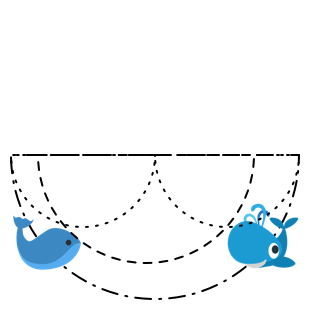# Erk Erk Erk

Calculus Level 5Below a depth of about 600 meters, sound travels at a speed that is dependent on the depth of the surface. Sounds actually travel faster the deeper one goes into the ocean, hence sound waves will travel faster by going downwards first.

Suppose that two whales, some distance apart, play at the surface of the sea. One whale emits a cry, which travels toward the other whale along an arc that goes down 7.5 kilometers (without hitting the ocean’s bottom). How long (in seconds) does the cry take to reach the other whale?

Assume that the speed of sound at depth D km is $\frac{ D + 72.5 } { 50 }$ kilometers per second (so the speed changes even at the surface, contrary to the initial statement).

This problem is shared by Gary Antonick.

×